The SEVERITY Procedure

Output Data Sets

Subsections:

PROC SEVERITY writes the OUTCDF=, OUTEST=, OUTMODELINFO=, and OUTSTAT= data sets when requested by their respective options. The data sets and their contents are described in the following sections.

OUTCDF= Data Set

The OUTCDF= data set records the estimates of the cumulative distribution function (CDF) of each of the specified model distributions and an estimate of the empirical distribution function (EDF).

If you specify BY variables, then the data are organized in BY groups and the data set contains variables that you specify in the BY statement. In addition, the data set contains the following variables:

<response variable>

value of the response variable. The values are sorted. If there are multiple BY groups, the values are sorted within each BY group.

_OBSNUM_

observation number in the DATA= data set. This is a sequence number that indicates the order in which the procedure accesses the observation; it does not necessarily reflect the actual observation number in the data set.

_EDF_

estimate of the empirical distribution function (EDF). This estimate is computed by using the EMPIRICALCDF= option that you specify in the PROC SEVERITY statement.

_EDF_STD

estimate of the standard error of EDF. This estimate is computed by using a method that is appropriate for the EMPIRICALCDF= option that you specify in the PROC SEVERITY statement.

_EDF_LOWER

estimate of the lower confidence limit of EDF for a pointwise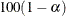% confidence interval, whereis the value of the EDFALPHA= option that you specify in the PROC SEVERITY statement (default is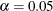). For an EDF estimate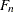that has standard error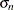, it is computed as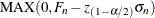, where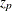is the pth quantile from the standard normal distribution.

_EDF_UPPER

estimate of the upper confidence limit of EDF for a pointwise% confidence interval, whereis the value of the EDFALPHA= option that you specify in the PROC SEVERITY statement (default is). For an EDF estimatethat has standard error, it is computed as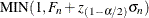, whereis the pth quantile from the standard normal distribution.

<distribution1>_CDF …<distributionD>_CDF

estimate of the cumulative distribution function (CDF) for each of the D candidate distributions, computed by using the final parameter estimates for that distribution. This value is missing if the parameter estimation process does not converge for the given distribution.

If you specify regression effects, then the reported estimates are from a mixture distribution. For more information, see the section CDF and PDF Estimates with Regression Effects.

If you specify truncation, then the data set contains the following additional variables:

<distribution1>_COND_CDF …<distributionD>_COND_CDF

estimate of the conditional CDF for each of the D candidate distributions, computed by using the final parameter estimates for that distribution. This value is missing if the parameter estimation process does not converge for the distribution. The conditional estimates are computed by using the method that is described in the section Truncation and Conditional CDF Estimates.

OUTEST= Data Set

The OUTEST= data set records the estimates of the model parameters. It also contains estimates of their standard errors and optionally their covariance structure. If you specify BY variables, then the data are organized in BY groups and the data set contains variables that you specify in the BY statement.

If you do not specify the COVOUT option, then the data set contains the following variables:

_MODEL_

_TYPE_

type of the estimates reported in this observation. It can take one of the following two values:

EST

point estimates of model parameters

STDERR

standard error estimates of model parameters

_STATUS_

status of the reported estimates. The possible values are listed in the section _STATUS_ Variable Values.

<Parameter 1> …<Parameter M>

M variables, named after the parameters of all candidate distributions, that contain estimates of the respective parameters. M is the cardinality of the union of parameter name sets from all candidate distributions. In an observation, estimates are populated only for parameters that correspond to the distribution that is indicated by the _MODEL_ variable. If _TYPE_ is EST, then the estimates are missing if the model does not converge. If _TYPE_ is STDERR, then the estimates are missing if covariance estimates cannot be obtained.

If you specify regression effects, then the estimate that is reported for the first parameter of each distribution is the estimate of the base value of the scale or log-transformed scale parameter. For more information, see the section Estimating Regression Effects.

<Regression Effect 1> …<Regression Effect K>

If your effect specification in the SCALEMODEL statement results in K regression effects, then the OUTEST= data set contains K regression variables. The name of each variable is formed by using the name of the effect and the names of the levels of the CLASS variables that the effect might contain. If the effect name or level names are too long, then the variable name is constructed by using partial effect name and integer identifiers for BY groups and CLASS variable levels. The label of the variable is more descriptive than the name of the variable. The variables contain estimates for their respective regression coefficients. If an effect is deemed to be linearly dependent on other effects for a given BY group, then a warning message is written to the SAS log and a special missing value of .R is written in the respective variable. If _TYPE_ is EST, then the estimates are missing if the model does not converge. If _TYPE_ is STDERR, then the estimates are missing if covariance estimates cannot be obtained.

<Offset Variable>

If you specify an OFFSET= variable in the SCALEMODEL statement, then the OUTEST= data set contains a variable that is named after the offset variable. If _TYPE_ is EST, then the value of this variable is 1. If _TYPE_ is STDERR, then the value of this variable is a special missing value of .F.

If you specify the COVOUT option in the PROC SEVERITY statement, then the OUTEST= data set contains additional observations that contain the estimates of the covariance structure. Given the symmetric nature of the covariance structure, only the lower triangular portion is reported. In addition to the variables listed and described previously, the data set contains the following variables that are either new or have a modified description:

_TYPE_

type of the estimates reported in this observation. For observations that contain rows of the covariance structure, the value is COV.

_STATUS_

status of the reported estimates. For observations that contain rows of the covariance structure, the status is 0 if covariance estimation was successful. If estimation fails, the status is 1 and a single observation is reported with _TYPE_=COV and missing values for all the parameter variables.

_NAME_

name of the parameter for the row of covariance matrix that is reported in the current observation.

OUTMODELINFO= Data Set

The OUTMODELINFO= data set records the information about each candidate distribution that you specify in the DIST statement. It contains the following variables:

_MODEL_

_DEPVAR_

name of the loss variable.

_DESCRIPTION_

descriptive name of the model. This has a nonmissing value only if the DESCRIPTION function has been defined for this model.

_VALID_

validity of the distribution definition. This has a value of 1 for valid definitions and a value of 0 for invalid definitions. If the definition is invalid, then PROC SEVERITY writes the reason for invalidity to the SAS log.

_PARMNAME1 ... _PARMNAMEM

M variables that contain names of parameters of the distribution model, where M is the maximum number of parameters across all the specified distribution models. For a given distribution with m parameters, values of variables _PARMNAMEj (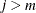) are missing.

OUTSTAT= Data Set

The OUTSTAT= data set records statistics of fit and model selection information. If you specify BY variables, then the data are organized in BY groups and the data set contains variables that you specify in the BY statement. The data set contains the following variables:

_MODEL_

_NMODELPARM_

number of parameters in the distribution.

_NESTPARM_

number of estimated parameters. This includes the regression parameters, if you specify any regression effects.

_NOBS_

number of nonmissing observations used for parameter estimation.

_STATUS_

status of the parameter estimation process for this model. The possible values are listed in the section _STATUS_ Variable Values.

_SELECTED_

indicator of the best distribution model. If the value is 1, then this model is the best model for the current BY group according to the specified model selection criterion. This value is missing if the parameter estimation process does not converge for this model.

Neg2LogLike

value of the log likelihood, multiplied by –2, that is attained at the end of the parameter estimation process. This value is missing if the parameter estimation process does not converge for this model.

AIC

value of the Akaike’s information criterion (AIC) that is attained at the end of the parameter estimation process. This value is missing if the parameter estimation process does not converge for this model.

AICC

value of the corrected Akaike’s information criterion (AICC) that is attained at the end of the parameter estimation process. This value is missing if the parameter estimation process does not converge for this model.

BIC

value of the Schwarz Bayesian information criterion (BIC) that is attained at the end of the parameter estimation process. This value is missing if the parameter estimation process does not converge for this model.

KS

value of the Kolmogorov-Smirnov (KS) statistic that is attained at the end of the parameter estimation process. This value is missing if the parameter estimation process does not converge for this model.

value of the Anderson-Darling (AD) statistic that is attained at the end of the parameter estimation process. This value is missing if the parameter estimation process does not converge for this model.

CVM

value of the Cra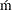er-von Mises (CvM) statistic that is attained at the end of the parameter estimation process. This value is missing if the parameter estimation process does not converge for this model.

_STATUS_ Variable Values

The _STATUS_ variable in the OUTEST= and OUTSTAT= data sets contains a value that indicates the status of the parameter estimation process for the respective distribution model. The variable can take the following values in the OUTEST= data set for _TYPE_=EST observations and in the OUTSTAT= data set:

0

The parameter estimation process converged for this model.

301

The parameter estimation process might not have converged for this model because there is no improvement in the objective function value. This might indicate that the initial values of the parameters are optimal, or you can try different convergence criteria in the NLOPTIONS statement.

302

The parameter estimation process might not have converged for this model because the number of iterations exceeded the maximum allowed value. You can try setting a larger value for the MAXITER= options in the NLOPTIONS statement.

303

The parameter estimation process might not have converged for this model because the number of objective function evaluations exceeded the maximum allowed value. You can try setting a larger value for the MAXFUNC= options in the NLOPTIONS statement.

304

The parameter estimation process might not have converged for this model because the time taken by the process exceeded the maximum allowed value. You can try setting a larger value for the MAXTIME= option in the NLOPTIONS statement.

400

The parameter estimation process did not converge for this model.

The _STATUS_ variable can take the following values in the OUTEST= data set for _TYPE_=STDERR and _TYPE_=COV observations:

0

The covariance and standard error estimates are available and valid.

1

The covariance and standard error estimates are not available, because the process of computing covariance estimates failed.